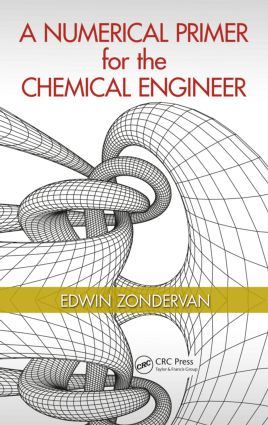A Numerical Primer for the Chemical Engineer

1st Edition

CRC Press

193 pages | 61 B/W Illus.

Purchasing Options:\$ = USD
Hardback: 9781482229448
pub: 2014-08-12
SAVE ~\$18.99
\$94.95
\$75.96
x
eBook (VitalSource) : 9780429189760
pub: 2014-08-12
from \$47.48

FREE Standard Shipping!

Description

Solve Developed Models in a Numerical Fashion

Designed as an introduction to numerical methods for students, A Numerical Primer for the Chemical Engineer explores the role of models in chemical engineering. Combining mathematical correctness (model verification) with numerical performance (model validation), this text concentrates on numerical methods and problem solving, rather than focusing on in-depth numerical analysis. It applies actual numerical solution strategies to formulated process models to help identify and solve chemical engineering problems.

Describe Motions with Accuracy

The book starts with a recap on linear algebra, and uses algorithms to solve linear equations, nonlinear equations, ordinary differential equations, and partial differential equations (PDEs). It includes an introductory chapter on MATLAB® basics, contains a chapter on the implementation of numerical methods in Excel, and even adopts MATLAB and Excel as the programming environments throughout the text.

The material addresses implicit and explicit schemes, and explores finite difference and finite volume methods for solving transport PDEs. It covers the methods for error and computational stability, as well as curve fitting and optimization. It also contains a case study chapter with worked out examples to demonstrate the numerical techniques, and exercises at the end of each chapter that students can use to familiarize themselves with the numerical methods.

A Numerical Primer for the Chemical Engineer lays down a foundation for numerical problem solving and sets up a basis for more in-depth modeling theory and applications. This text addresses the needs of senior undergraduates in chemical engineering, and students in applied chemistry and biochemical process engineering/food process engineering.

The role of models in chemical engineering

Introduction

The idea of a model

Model building

Model analysis

Model solution strategies

Summary

Exercises

Errors in computer simulations

Introduction

Significant digits

Round off and truncation errors

Break errors

Loss of digits

Ill conditioned problems

(Un-)stable methods

Summary

Exercises

Linear equations

Introduction

MATLAB

Linear systems

The inverse of a matrix

The determinant of a matrix

Useful properties

Matrix ranking

Eigenvalues and eigenvectors

Spectral decomposition

Summary

Exercises

Elimination methods

Introduction

MATLAB

Gaussian elimination

LU factorization

Summary

Exercises

Iterative methods

Introduction

Laplace’s equation

LU factorization

Iterative methods

The Jacobi method

Example for the Jacobi method

Summary

Exercises

Nonlinear equations

Introduction

Newton’s method D

Newton’s method D

Reduced Newton step method

Quasi Newton’s method

Summary

Exercises

Ordinary diﬀerential equations

Introduction

Euler’s method

Accuracy and stability of Euler’s method

The implicit Euler method

Stability of the implicit Euler method

Systems of ODE’s

Stability of ODE systems

Stiﬀness of ODE systems

Higher order methods

Summary

Exercises

Partial diﬀerential equations 1

Introduction

Types of PDE’s

The method of the lines

Stability

Summary

Exercises

Partial diﬀerential equations 2

Introduction

Transport PDE’s

Finite Volumes

Discretizing the control volumes

Transfer of heat to ﬂuid in a pipe

Simulation of the heat PDE

Summary

Exercises

Data regression and curve ﬁtting

Introduction

The least squares method

Residual analysis

ANOVA analysis

Conﬁdence limits

Summary

Exercises

Optimization

Introduction

Linear programming

Nonlinear programming

Integer programming

Summary

Exercises

Basics of Matlab

Introduction

The Matlab user interface

The array structure

Basic calculations

Plotting

Reading and writing data

Functions and m-ﬁles

Repetitive operations

Numerical methods in Excel

Introduction

Basic functions in Excel

The Excel solver

Solving nonlinear equations in Excel

Diﬀerentiation Excel

Curve ﬁtting in Excel

Case studies

Introduction

Modeling a separation system

Modeling a chemical reactor system

PVT behavior of pure substances

Dynamic modeling of a distillation column

Dynamic modeling of an extraction cascade (ODE’s)

Distributed parameter models for a tubular reactor

Modeling of an extraction column

Fitting of kinetic data

Fitting of NRTL model parameters

Optimizing a crude oil reﬁnery

Planning in a manufacturing line

Bibliography

Edwin Zondervan obtained a bachelor degree in chemical engineering at Noordelijke Hogeschool Leeuwarden. In November 2003 he graduated from Groningen University with an M.Sc. in chemical engineering. In November 2007 he obtained his Ph.D. at Groningen University. Zondervan has published in several journals. He currently acts as a reviewer for the Journal of Membrane Science, Computers and Chemical Engineering, Journal of Environmental Management, Industrial Engineering & Chemistry Research, Separation and Purification Technology, and Recent Patent in Chemical Engineering. From 2007 to 2010 he has worked as an assistant professor at Eindhoven University. He recently moved to the Polymer Reaction Engineering group.